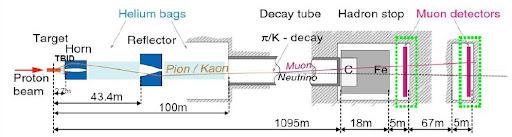### Waiting superluminal neutrinos: from Maxwell to Einstein(CNGS device)
$\vec \nabla \cdot \vec E = \frac{\rho}{\varepsilon_0}$ $\vec \nabla \cdot \vec B = 0$ $\vec \nabla \times \vec E = - \frac{\partial \vec B}{\partial t}$ $\vec \nabla \times \vec B = \mu_0 \vec J + \mu_0 \varepsilon_0 \frac{\partial \vec E}{\partial t}$ In 1861 James Maxwell published the first (of four) part of the paper On Physical Lines of Force, in which he stated his famous equations on electromagnetic field. One of the most important thing about these equations is that they are not invariant under Galilei's transformations! But, if we search the symmetry transformation of the equations, we find Lorentz transformations: $\begin{cases} t' &= \gamma \left( t - \frac{v x}{c^2} \right) \\ x' &= \gamma \left( x - v t \right)\\ y' &= y \\ z' &= z \end{cases}$ where $\gamma = \frac{1}{\sqrt{1-\frac{v^2}{c^2}}}$.
From these transformations, discovered in 1887(1, 2), we can extract the physics of special relativity, thanks to Albert Einstein. So, if we want tosubstitute c with the alleged OPERA's neutrinos speed, we must conclude that the new boson particle of electromagnetic field is the neutrino! In this sense I say that special relativity is right: our universe and our observations are based on electromagnetic field, so if OPERA results will be verified, we probably think to:
1. change the weak interaction(3);
2. study an eventually quantum interaction between neutrinos and space time(4);
3. imagine a new field exclusively for neutrinos(5);
4. other way that in this moment I cannot imagine(6)!
This is the science, people: we find data, we search interpretation, we quest for confirmation, we calculate mathematical explenation, and only at this point we write text books. But, and this is the most important idea, we don't reject the old theories: Newton and Galilei's theories are today right, but we must substitute them in quantum world and to a cosmo's schale. And the destiny of model standard and Einstein's relativity is the same, and our task is find their limits.
(1) O'Connor, John J.; Robertson, Edmund F., A History of Special Relativity
(2) Sinha, Supurna (2000), Poincaré and the Special Theory of Relativity, Resonance 5: 12–15
(3) Yesterday I say that the changes are only at higher orders. I think that is a good idea, because we verified weak interaction very well, but change the higher orders is not a trivial calculation!
(4) For example we can imagine that neutrinos are the particle with the most strong interaction with an eventually quantum gravity. So, if OPERA results will be confirmed from an other experiment, we could suppose that these results are the first signature of the quantum gravity.
(5) Recently I followed an interesting seminar about neutrinos and dark matter. Valeria Pettorino, the speaker, presented an interesting model in which she propose, with his research group, a new fifth interaction between darkmatter and neutrinos, that are the only particles in the universe to interact to dark matter (for example, you can read the followin preprint).
(6) The last idea that I want propose in these footnotes is probably the most simple (and probably most sci-fi!): at CERN we would recreate the Big Bang conditions, so we could imagine that OPERA's neutrinos are simply neutrinos from Big Bang. Indeed we don't remember that c is the light speed now, but we don't know if in the past c was different.
And don't forget: in this post I try to explane the science path and I try to play with the possibilities